## Introduction

The thermal conductivity of materials is a key property that is attracting much interest both for its role and relevance to thermoelectric waste heat recovery, and for the scientific questions and technological opportunities that are arising in the field of low-dimensional materials (see, for example, refs 1, 2 for a review). When dimensionality is reduced or the relevant sizes reach microscopic scales (from tens of nanometers to tens of microns), the knowledge developed in the past on conventional bulk crystals becomes insufficient, and novel properties emerge. The prototypical two-dimensional (2D) material, graphene, has the highest known thermal conductivity (even if there is to-date no complete consensus on its actual value1), and unexpected phenomena have been observed experimentally, with, for example, a size dependence in the thermal conductivity for samples reaching the micron scale3. This is consistent with the theoretical prediction that heat carriers in graphene can propagate for distances up to the millimetre scale4. The origin of such behaviour is under active investigation and much debated, with arguments centring around dimensionality effects3,5,6 and the reduction of the scattering phase space7.

It has been noted that for graphene and boron nitride4,7,8,9 it is essential to consider the exact solution of the Boltzmann transport equation (BTE) when studying thermal conductivity, and that the widely used single-mode relaxation time approximation (SMA; where individual phonons thermalize independently, without collisions repopulating them) fails in describing correctly thermal transport. This failure arises because the simultaneous interaction of all phonon populations is crucial, and such collective behaviour4,10 can lead to the emergence of composite excitations as the leading heat carriers.

Such collective behaviour is driven by the dominance of normal (that is, heat-flux conserving) phonon scattering events, which allow the phonon gas to conserve to a large extent its momentum before other resistive scattering mechanisms can dissipate it away. A phonon gas in such state is said to be in the hydrodynamic regime, because of the similarity with the case of an ideal gas where particles scatter without dissipating momentum. Phonons in the hydrodynamic regime can, under specific conditions, form packets that alter the typical diffusive behaviour of heat and make it propagate as a damped wave, giving origin to the phenomenon of second sound, where a localized heating perturbation generates two sound wavefronts when probed at a certain distance11,12. This phenomenon has not been extensively studied, as to-date it was possible to observe it only in few materials at cryogenic temperatures (10 K or less): solid helium13, sodium fluoride11,14, bismuth12, strontium titanate15,16 (theoretically it is also been hypothesized for diamond17).

In this work, we study the lattice thermal conductivity of graphene, graphane, boron nitride, fluorographene and molybdenum disulphide by solving the linearized BTE using a variational approach devised by some of us18,19 that is particularly robust in converging to the exact solution of the BTE under all possible conditions (iterative solutions have limited domains of convergence20,21,22 and this is particularly challenging in 2D materials4,7, because of the collective nature of the excitations). In addition, all calculations are parameter free, as scattering rates and lifetimes for phonon–phonon interactions and for isotopic elastic scattering are computed using phonon frequencies and phonon–phonon interactions from density-functional pertubation theory23,24,25,26,27,28, in a reciprocal-space formulation able to deal with any arbitrary wavevector29. Isotopic concentrations of the five materials use the natural abundances (see the Methods section for details).

Remarkably, we show that all five materials considered fall into the hydrodynamic regime even at room temperature or above it, and we highlight the persistence of second sound in graphene, graphane and boron nitride at temperatures well-above cryogenic. In graphene at room temperature, second sound propagates over distances of the order of 1 μm, hinting at the possibility of devising thermal devices based on coherent propagation30. Last, we also provide validation for the Callaway model of thermal conductivity, developed for heat transport at very low temperatures, and shown here to be valid for these 2D materials up to room temperature and above.

## Results

### Boltzmann transport equation

The thermal conductivity k is the quantity, which relates the heat flux Q with a gradient of temperature T, so that Q=−kT; for simplicity, the thermal conductivity, which in general is a tensor, is written as a scalar property, as we are considering only the in-plane conductivity, that is, isotropic. To express k as a function of microscopic quantities, one introduces the out-of-equilibrium phonon distribution nv (ν=q, s labels collectively the Brillouin zone wavevectors and phonon branches). For small perturbations, nv is linearized around the Bose–Einstein thermal equilibrium distribution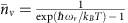so that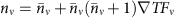, and all deviations from equilibrium are expressed by the set of functions Fv. Using the microscopic expression of the heat flux31,32, k can be expressed in terms of microscopic quantities as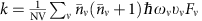, where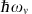is the energy of the phonon ν, υν is the projection of the phonon group velocity on the direction parallel to T and NV is the volume of the crystal. Thus, k depends on harmonic quantities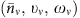, which can be computed routinely and inexpensively even from first-principles23,24, and the challenge is to find the Fv deviations from equilibrium.

To obtain nv, we solve the linearized BTE18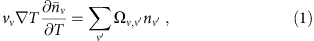where the scattering operator is represented by a matrix of scattering rates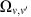acting on the phonon populations nv. We focus here mostly on intrinsic processes and isotopic disorder, so the scattering matrix is built using three-phonon processes and isotopic elastic rates as detailed in refs 19, 26; extrinsic sources of scattering (typically, finite sizes) are discussed in connection with the Poiseuille thermal conductivity regime.

For simplicity, the BTE is often solved in the SMA, which relies on the assumption that heat-current is dissipated every time a phonon undergoes a scattering event18. Therefore, the phonon distribution relaxes towards thermal equilibrium at a rate which is given by the phonon lifetime τv, the average time between phonon scattering events at equilibrium. The scattering operator is thus approximated as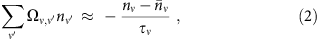and the SMA thermal conductivity depends directly on the individual phonon lifetimes: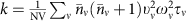.

The comparison between the SMA thermal conductivity as a function of temperature and the exact solution of the BTE is reported in Fig. 1a for all five materials considered here, and the qualitative failure of the SMA at all temperatures becomes immediately apparent. This failure has already been highlighted, for example, for the case of graphene and boron nitride, using either first-principles scattering rates for graphene4,7 or empirical potentials8,9. As expected, we find that such failure occurs also in graphane and molybdenum disulphide, and, by a smaller amount, in fluorographene, making it common in 2D materials. Interestingly, errors affect also qualitative predictions: for example, the SMA solution predicts graphane to have a conductivity larger than graphene at room temperature.

### Poiseuille and Ziman hydrodynamics

To make further progress, it is very instructive to inspect the scattering rates for the different processes involved, that is, three-phonon processes (divided into normal (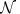) and Umklapp (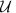) events) and isotopic scattering (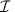). Forprocesses, total momentum is conserved and all three phonon wavevectors belong to the first Brillouin Zone, whereas inprocesses momentum is not conserved, and the sum of the three wavevectors corresponds to a non-zero reciprocal lattice vector. Such distinction is key for heat transport, as it has been long known18 thatprocesses do not actively dissipate heat-current, as a consequence of energy and momentum conservation, andprocesses act as the source of intrinsic dissipation.

We show in Fig. 2 the average linewidths for all these processes, defined as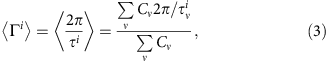where i labels either,orprocesses and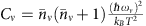is the specific heat of the phonon mode ν (this weight is the same appearing in the second-sound velocity shown later). Conventionally, it is expected thatevents become dominant only at very low temperatures, whenprocesses freeze out18, and even then only provided thatrates or other extrinsic sources of scattering are negligible. Quite surprisingly, it is seen here that not onlyprocesses are very relevant, as argued in refs 8, 29, but also that they represent the dominant scattering mechanisms in these 2D materials at any temperature (with the exception of natural-abundance molybdenum disulphide and boron nitride, whereis comparable tobecause of the large isotopic disorder of molybdenum and boron).

The predominance of normal scattering processes identifies the regime(s) under which the phonon gas is defined to be in the hydrodynamic conditions mentioned above. We nevertheless underscore that, even if the momentum-conserving nature ofprocesses prevents them from dissipating heat-current, they still affect the total thermal conductivity by altering the out-of-equilibrium phonon distribution. Last, we highlight that the failure of the SMA in these conditions is a rather conceptual one, as it is the assumption that heat flux is dissipated at every scattering event that becomes invalid. Instead, given that most processes are normal, heat flux is just being shuttled between phonon modes.

To compare these 2D materials with conventional solids, we summarize in Table 1 the different regimes of phonon transport according to the nomenclature of Guyer33. In the ballistic regime, the dominanant scattering events are extrinsic (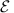), and are typically due to the finite size of the sample (line defects, surfaces, grain boundaries); in the Poiseuille regime, normal () processes dominate, and the heat flux is dissipated byevents (this is the first hydrodynamic regime where the gas of phonons is ‘normal’ but it feels the ‘walls’ of the container). In the Ziman regime,events still dominate, but the heat flux is now dissipated by resistive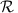scattering (either Umklapp () or isotopic (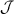))—in this regime, the ‘walls’ have become irrelevant for the normal gas. Finally, in the kinetic regime intrinsic resistive processes(again, typicallyor) have the highest linewidth, the sample size is much larger than all typical mean free paths andevents have become negligible.

It becomes instructive then to compare graphene, taken as reference, with a typical three-dimensional solid, like silicon or germanium. We show in Fig. 3 the thermal conductivity of graphene both in the case in which we have an infinite sample (k) or a finite ribbon (here of width L=100 μm, kL), with an extrinsic scattering rate given by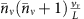(ref. 22), considering this also in the ballistic limit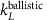obtained by removing all the internal sources of scattering,and, but preserving the extrinsic scattering. The comparison ofwith the exact thermal conductivity kL in a ribbon of width L shows that the ballistic regime is not a good approximation to kL. In fact, the introduction ofscattering events, that is, hydrodynamic conditions, enhances the thermal conductivity well above the ballistic limit. As the thermal conductivity grows with temperature, graphene is in the Poiseuille regime, whereevents facilitate the heat flux, and extrinsic sources of scattering dissipate it (the term Poiseuille comes from an analogy33 between the flow of phonons in a material with the flow of a fluid in a pipe). The thermal conductivity though does not grow indefinitely with temperature, because of the limit imposed by the intrinsic thermal conductivity of an infinite sheet k. Thus, there is a thermal conductivity peak, and for higher temperatures, the heat flux is mostly dissipated by intrinsic sources (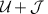). As theprocesses have the largest linewidth at any temperature, the regime at temperatures higher than the peak is still hydrodynamic, but it shifts to Ziman, where the intrinsic resistive processesset the typical length scales, and the boundaries become irrelevant. Last, if the intrinsic events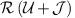had the largest linewidth, the thermal conductivity would be in the kinetic regime: this is the regime of conventional materials at ordinary temperatures, but it is not ever reached in these 2D cases.Figure 3: Thermal transport regimes in two- and three-dimensional (3D) materials.

In conclusion, the two major differences between these 2D materials and a conventional solid are (i) in the temperature scales involved (the non-kinetic regimes in a conventional solid are all condensed in narrow energy window around cryogenic conditions, and hydrodynamic regimes may not be present at all), and (ii) in the fact that even at the highest temperatures normal processes remain dominant, restricting conductivities to just the hydrodynamic regimes of Poiseuille and Ziman.

### Callaway’s model

Callaway’s model is an approximation to the scattering matrix that was originally developed in order to describe the thermal conductivity of germanium at very low temperatures (20 K or so)34. It had previously been shown by Klemens35 that the most probable boson distribution in a system where momentum is conserved is given by the drifting distribution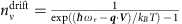, where V is a Lagrange multiplier enforcing momentum conservation. Therefore,processes will tend to relax the phonon population nv towards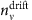. Instead, resistive processes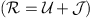tend to relax the phonon populations towards local thermal equilibrium (that is, the Bose–Einstein distribution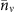), just as described by the SMA. Therefore, Callaway approximated the scattering operator as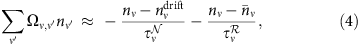where the Matthiessen rule (that is, arithmetic sum of the scattering rates for independent events) is used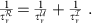Asscattering rates are very large, an out-of-equilibrium distribution will mostly decay first into the drifting distribution, and from this state it will relax towards static equilibrium. The results for the thermal conductivity in Callaway’s approximation are shown in Fig. 1b, and compared again against the results of the exact solution of the BTE (more details on the expression of Callaway’s thermal conductivity can be found in the Supplementary Note 1 and Supplementary Fig. 1). It can be seen that the model broadly reproduces the exact solutions, even if, for example, in fluorographene it overestimates the exact results, or in molybdenum disulphide underestimates them. So, even more than providing simple but fairly accurate estimates of the phonon distribution without the need of solving the BTE, the value of the model is in the clear physical insight it offers on the microscopic nature of heat transport, allowing us to conclude not only that the dominant presence ofprocesses at all temperatures characterizes heat transport in these materials, but also that the distribution function can be broadly described as a drifting distribution according to the prescriptions of Callaway and Klemens34,35.

### Second sound

Materials in hydrodynamic regimes can host second sound11,12,13,14,15,16, and is thus interesting to discuss its existence or characteristics in two dimensions. We define second sound, following ref. 36, as the propagation of heat in the form of a damped wave. In a conventional material, one expects only diffusive propagation; it is the ‘second’ heat-wave transport that gives the name to this phenomenon.

In principle, more than one microscopic mechanism could lead to the formation of second sound36; the common requirement is that a mechanism exists, which is causing a slow decay of the heat flux. This can indeed happen in case of a large presence ofevents. As mentioned before, the conservation of momentum drives nv towards, and once this drifting distributionhas accumulated, heat propagates as a wave that is eventually damped on longer timescales by the resistive processes that relax the phonons to the equilibrium Bose–Einstein distribution, and determine diffusive heat transport at longer lengths.

To observe second sound, the out-of-equilibrium distribution needs to be in the form and well described by the drifting distribution. We have already shown in the previous section that its introduction in the scattering operator provides a good estimate of the thermal conductivity. To see whether the out-of-equilibrium distribution closely resembles the drifting one, we first expand the drifting distribution in a Taylor series, so that it is simply proportional to the projection of the wavevector parallel to the temperature gradient (see Supplementary Note 2). Then, we define the drifting component as:which is equal to one if the out-of-equilibrium distribution is equal to the drifting distribution. We plot this quantity for all materials considered in Fig. 4 (as a percentage), and we observe that more than 40% of the phonon distribution of graphene at room temperature is determined by the drifting distribution, and that this ratio grows at lower temperatures; this ratio is slightly smaller in all other materials, falling below 30% for fluorographene and 10% molybdenum disulphide. Therefore, in graphene, graphane and boron nitride, a large fraction of the heat flux is carried by the drifting distribution (which is a good approximation of nv for the study of the heat flux propagation), whereas in fluorographene and molybdenum disulphide, the drifting distribution is a worse descriptor of the out-of-equilibrium distribution, as also hinted by the reduced effectiveness of the Callaway model.

When the drifting distribution is a good approximation to the out-of-equilibrium distribution, the material will display second sound. The second-sound heat wave is characterized by a velocity vss and a relaxation time τss that define a second-sound length λss=vss τss, that is, the characteristic distance that the heat wave propagates before decaying. It is possible to show (details are reported in the Supplementary Note 3) that, approximating the out-of-equilibrium distribution with the drifting distribution and using the Callaway approximation for the scattering operator, the BTE can be rewritten as a wave-like equation for the temperature profile, characterized by the following quantities: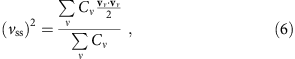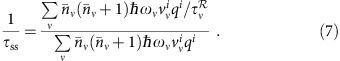The second-sound relaxation times—reported in the Supplementary Fig. 2—indicate that the energy flux dissipation decays on average on a time scale of the order of hundred picoseconds at room temperature for the three materials of higher conductivity. The second-sound velocities depend only on harmonic properties, and can be easily computed—they are reported in the Supplementary Fig. 3 as a function of temperature, and compared with the average velocity of acoustic phonons. We find that the different vss typically lay in between the larger average velocities of the longitudinal and tranverse acoustic branches, and the much lower average velocity of the out-of-plane acoustic mode.

The propagation lengths λss=τss vss for the second-sound wave for graphene and graphane reach the micron scale, even at room temperature, whereas boron nitride is characterized by second-sound lengths of a fraction of a micron (the values of λss for molybdenum disulphide and fluorographene are shown in Fig. 5 for completeness, even if those materials do not host second sound). At lower temperatures, whereprocesses tend to freeze and onlyare present, damping of the second sound becomes less effective and the heat wave propagates over longer distances.

As mentioned, the presence of second sound has been verified experimentally in the past11,12,13,14,15 in 3D materials at cryogenic temperatures, and it is conveniently studied as the response of a material to a temperature pulse. One should then be able to observe at a distance standard pulses due to the diffusive propagation by the longitudinal, tranverse and out-of-plane acoustic modes; in addition, the second-sound signature will appear as a further peak due to the formation of the drifting distribution. One should also always consider the size of the experimental setup, as this will affect phonons, which travel ballistically, that is, without scattering between the heat source and the detector, and that would diminish the component of heat travelling in the other modes. Their effect on second sound should be negligible as long as the distance between pump and probe is larger than λss. Finally, let us note that the estimate of λss is obtained here as a statistical average, and thus we cannot exclude the existence of tails of the second-sound mode propagating at much longer distances.

## Discussion

We have investigated heat transport in some key 2D materials—graphene, boron nitride, molybdenum disulphide and the functionalized derivatives graphane and fluorographene. We have found that momentum-conserving normal processes are the dominant scattering mechanisms at all temperatures, requiring an exact solution of the linearized BTE for qualitative and quantitative accuracy, and confirming that graphene provides the highest thermal conductivity among all materials considered. Heat transport in all cases falls into the conditions of Poiseuille and Ziman hydrodynamics (below and above the conductivity peak, respectively), never reaching the ‘ordinary’ conditions of diffusive transport even at very high temperatures. The Callaway model, developed originally for the study of heat transport in conventional three-dimensional solids in the hydrodynamic regimes that could arise at cryogenic temperatures, provides a broadly accurate description of heat transport in two dimensions at all temperatures. Moreover, its microscopic picture of two relaxation time scales, where the out-of-equilibrium distribution accumulates first into a momentum-conserving drifting distribution before relaxing into the final Bose–Einstein equilibrium, provides both microscopic insight and understanding. Graphene, graphane and boron nitride all display second sound, that is, a component in heat transport that behaves as a damped wave and is not described by diffusive propagation, with length scales that reach 1 μm for graphene at room temperature.

## Methods

### First-principles simulations

Second- and third-order force constants have been calculated using density-functional perturbation theory23,27,28,37 as implemented in the Quantum ESPRESSO distribution38, using the local-density approximation, norm-conserving pseudopotentials from the PSlibrary39 and a plane-wave cutoff of 90 Ry. The electronic-structure calculations for graphene (G), boron nitride (BN), fluorographene (CF) and graphane (CH) use a Gamma-centred Monkhorst-Pack Brillouin zone sampling of 24 × 24 × 1 k-points, and 16 × 16 × 1 k-points for MoS2. All systems are simulated in a supercell geometry, relaxing the in-plane lattice parameter a to equilibrium, and with interlayer distances of 7 Å for all materials except MoS2, where it is taken to be 18 Å. For graphene, that is a semimetal, we use a Methfessel-Paxton cold smearing of 0.02 Ry. For all materials, but not for MoS2, the harmonic and anharmonic force constants have been computed on meshes of 16 × 16 × 1 and 4 × 4 × 1 q-points in the Brillouin zone, respectively, whereas for MoS2 they have been computed on 16 × 16 × 1 and 6 × 6 × 1, respectively.

### Thermal conductivity simulations

The BTE is solved using the variational method of ref. 19, finding well-converged results for a mesh of 128 × 128 × 1 q-points and a Gaussian broadening of 10 cm−1, except for the case of MoS2, where a mesh of 140 × 140 × 1 q-points and a broadening of 1 cm−1 has been used. The isotopic concentrations are chosen to be the natural ones40: hydrogen of 99.9885% 1H, 0.0115% 2H; carbon of 98.93% 12C, 1.07% 14C; boron of 19.9% 10B, 80.1% 11B, nitrogen of 99.632% 14N, 0.368% 15N; fluorine of 100% 19F; sulphur of 94.93% 32S, 0.76 33S, 4.29 34S, 0.02% 36S; molybdenum of 14.84% 92Mo, 9.25% 94Mo, 15.92% 95Mo, 16.68% 96Mo, 9.55% 97Mo, 24.13% 98Mo, 9.63% 100Mo.

The numerical results presented in the text are normalized by the volume of the unit cell of the crystal; the thickness in the perpendicular direction is chosen as the experimental one of the corresponding 3D material, using c/a ratios of 1.367 for graphene and graphane, 1.317 for boron nitride, 1.206 for fluorographene and 1.945 for MoS2.

All calculations have been managed using the AiiDA materials’ informatics platform41.